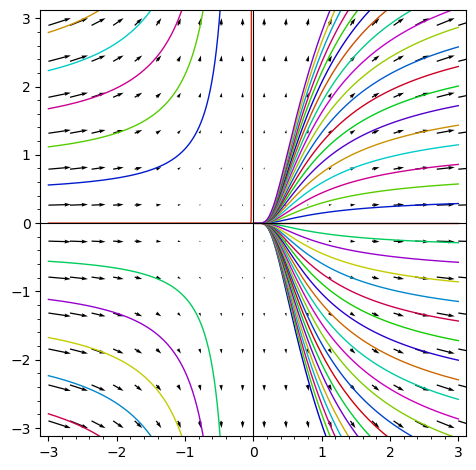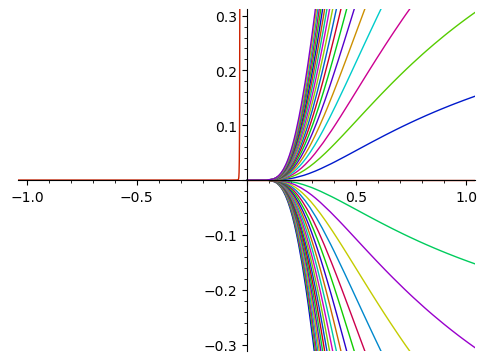# differential equationhi

we are discovering Sagemaths and trying to solve and graph a diff equation x^2*y'=y

we tryed to agglomerate two sheets of code :

• the one which solves (and works alone)

• the other one which draws (and works alone)

but together they dont work (maybe because of _C ?)

any help welcome

Vinz+Erw

x = var('x'); y = function('y')
yprime=diff(y(x),x)
EqDf = (x**2)*yprime==y(x)
g(x)=desolve(EqDf, [y(x),x])
g(x)

#dessin = plot([g(x) for _C in srange(-8, 8, 0.4)], (x, -3, 3))
dessin = plot(g(x), (x, -3, 3))

y = var('y')
dessin += plot_vector_field((x^2, y), (x,-3,3), (y,-5,5))

dessin.show(aspect_ratio = 1, ymin = -3, ymax = 3)

edit retag close merge delete

Sort by » oldest newest most votedIndeed, if you want to plot $g(x)$, the expression $g(x)$ should evaluate to a number when $x$ is a number. You correctly determined that the presence of the symbolic variable _C (introduced by the ODE solver) spoils the fun. Your attempt [g(x) for _C in srange(-8, 8, 0.4)] however creates just a list of copies of $g(x)$, without doing any substitution (that you intended). To do the subtitution, you can write e.g. [g(x).subs({var('_C') : _C}) for _C in srange(-8, 8, 0.4)]. Then it works:more

As observed in the question, the solution contains a parameter _C.

That parameter does not get declared globally, so we need to do that before we can use it.

Here is one way using subs.

Define the variable, the function, the differential equation.

sage: x = SR.var('x')
sage: y = function('y')
sage: yprime = diff(y(x), x)
sage: de = (x^2)*yprime == y(x)
sage: de
x^2*diff(y(x), x) == y(x)


Solve.

sage: g(x) = desolve(de, [y(x), x])
sage: g
x |--> _C*e^(-1/x)


Declare the parameter as a symbolic variable.

sage: _C = SR.var('_C')


Plot solutions for a range of values of the parameter.

sage: dessin = plot([g(x).subs({_C: c}) for c in srange(-8, 8, 0.4)], (x, -3, 3))
sage: dessin.show(xmin=-1, xmax=1, ymin=-0.3, ymax=0.3)
Launched png viewer for Graphics object consisting of 80 graphics primitives
`more